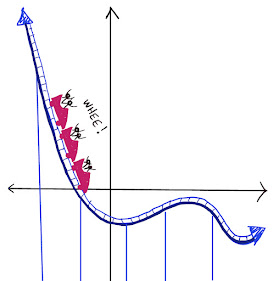## Wednesday, October 28, 2015

### Four Paths Problem

For the solution, click "Read More" below.

#### It seems counterintuitive, but the first three paths are all the same size.  If the squares are all unit squares, then the lengths of each of the first 3 paths in 2 units.   One way to understand this is that if we have a stair-step pattern made of right-angled turns, then the sum of the lengths of all of the horizontal lines must 1 and the sum of the lengths of all of the vertical lines must 1 -- no matter how many "steps" there are in the path.  The diagonal, on the other hand, is shorter.  Its length is the square root of 2 units.